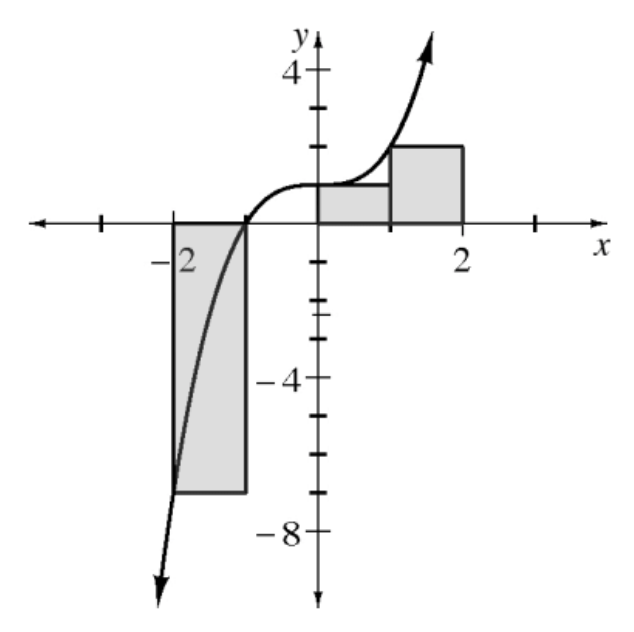Home > APCALC > Chapter 1 > Lesson 1.4.2 > Problem1-160

1-160.

The function $y = x^3 + 1$ is graphed at right, along with four left endpoint rectangles which approximate the area under the curve from $x = -2$ to $x = 2$.

1. Why does it look like there are only three rectangles?

What happens if the left endpoint is on the $x$-axis?

Since $f(−1) = 0$, the height of the rectangle is $0$.

2. Recall that area under the $x$-axis is negative, while area above the $x$-axis is positive. Approximate the area under the curve for $−2 ≤ x ≤ 2$ using these four rectangles.

Sum the areas of all four rectangles together. Remember to treat a rectangle's area as negative if it is below the $x$-axis.